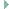div.h File Reference

## Detailed Description

`#include <assert.h>`
`#include <stdint.h>`Include dependency graph for div.h:This graph shows which files directly or indirectly include this file:

Go to the source code of this file.

 #define DIV_H_INV_15625_32   0x431bde83ul Approximation of (2**l)/d for d=15625, l=12, 32 bits. #define DIV_H_INV_15625_64   0x431bde82d7b634dbull Approximation of (2**l)/d for d=15625, l=12, 64 bits. #define DIV_H_INV_15625_SHIFT   12 Required shifts for division by 15625, l above. uint64_t _div_mulhi64 (const uint64_t a, const uint64_t b) static uint64_t div_u64_by_15625 (uint64_t val) Integer divide val by 15625, 64 bit version. More... static uint32_t div_u64_by_125 (uint64_t val) Integer divide val by 125. More... static uint64_t div_u64_by_1000000 (uint64_t val) Integer divide val by 1000000. More... static uint32_t div_u32_by_15625div512 (uint32_t val) Divide val by (15625/512) More... static uint64_t div_u64_by_15625div512 (uint64_t val) Divide val by (15625/512) More... static uint32_t div_u32_by_44488 (uint32_t val) Integer divide val by 44488. More... static uint32_t div_u32_mod_44488 (uint32_t val) Modulo 44488. More...

## ◆ div_u32_by_15625div512()

 static uint32_t div_u32_by_15625div512 ( uint32_t val )
inlinestatic

Divide val by (15625/512)

This is used to quantize a 1MHz value to the closest 32768Hz value, e.g., for timers.

The algorithm uses the modular multiplicative inverse of 15625 to use only multiplication and bit shifts to perform the division.

The result will be equal to the mathematical expression: floor((val * 512) / 15625)

Parameters
 [in] val dividend
Returns
(val / (15625/512))

Definition at line 130 of file div.h.

## ◆ div_u32_by_44488()

 static uint32_t div_u32_by_44488 ( uint32_t val )
inlinestatic

Integer divide val by 44488.

Parameters
 [in] val dividend
Returns
(val / 44488)

Definition at line 164 of file div.h.

## ◆ div_u32_mod_44488()

 static uint32_t div_u32_mod_44488 ( uint32_t val )
inlinestatic

Modulo 44488.

Parameters
 [in] val dividend
Returns
(val % 44488)

Definition at line 175 of file div.h.

## ◆ div_u64_by_1000000()

 static uint64_t div_u64_by_1000000 ( uint64_t val )
inlinestatic

Integer divide val by 1000000.

Parameters
 [in] val dividend
Returns
(val / 1000000)

Definition at line 111 of file div.h.

## ◆ div_u64_by_125()

 static uint32_t div_u64_by_125 ( uint64_t val )
inlinestatic

Integer divide val by 125.

This function can be used to convert uint64_t microsecond times (or intervals) to milliseconds and store them in uint32_t variables, with up to ~50 days worth of milliseconds ((2**32*1000) -1). Use e.g., ms = div_u64_by_125(microseconds >> 3)

Precondition
val <= 536870911999 ((2**32 * 125) -1)
Parameters
 [in] val dividend
Returns
(val / 125)

Definition at line 91 of file div.h.

## ◆ div_u64_by_15625()

 static uint64_t div_u64_by_15625 ( uint64_t val )
inlinestatic

Integer divide val by 15625, 64 bit version.

Parameters
 [in] val dividend
Returns
(val / 15625)

Definition at line 70 of file div.h.

## ◆ div_u64_by_15625div512()

 static uint64_t div_u64_by_15625div512 ( uint64_t val )
inlinestatic

Divide val by (15625/512)

This is used to quantize a 1MHz value to the closest 32768Hz value, e.g., for timers.

Parameters
 [in] val dividend
Returns
(val / (15625/512))

Definition at line 144 of file div.h.